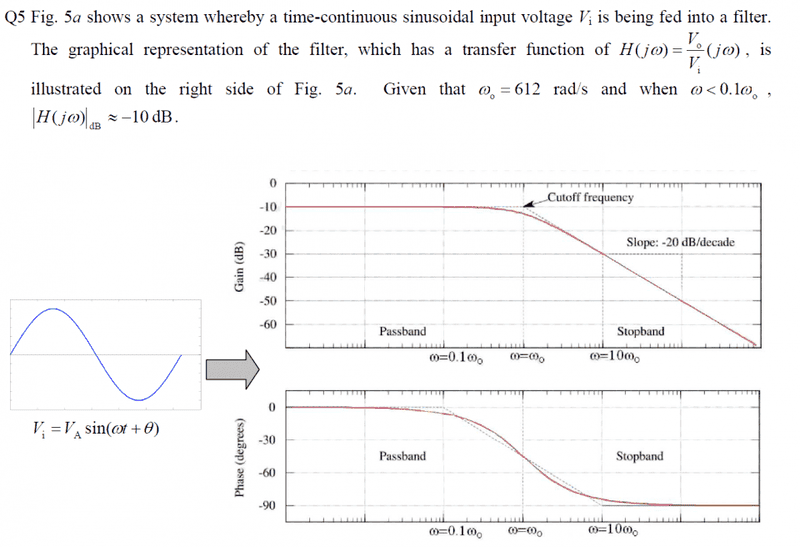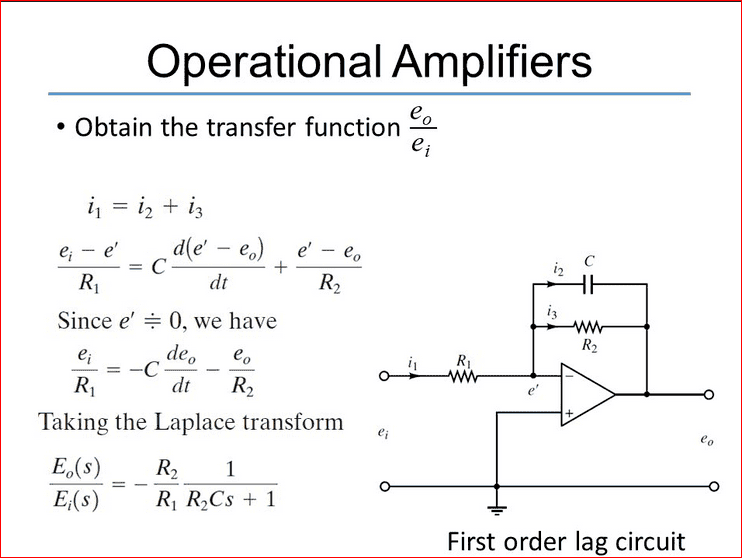Determine the transfer function of the system H(jw)
why the answer is not 1/[1+(jw/612)] but equal to 0.318/[1+(jw/612)]?

What happens if you insert w=0 into the equation? Can you determine the remaining value?

What happens if you insert w=0 into the equation? Can you determine the remaining value?
to which equation?Vi=Va sin(wt+θ)?
i find that 0.318 is 1/π where the π come from?

You have asked for the transfer function - thus, I mean H(jw), of course.

You have asked for the transfer function - thus, I mean H(jw), of course.
but the equation of H(jw)=1/[1+jwRC] (from my lecture notes) 1/Rc=w0 (for w<0.1w0), hence i did not where 0.318 come from

The magnitude plot shows for small w values (down to w=0) a level of -10dB. Can you calculate the corresponding absolute value?
Then, you must compare this result with both transfer function for the case w=0. Which one is valid?

The magnitude plot shows for small w values (down to w=0) a level of -10dB. Can you calculate the corresponding absolute value?
Then, you must compare this result with both transfer function for the case w=0. Which one is valid?
20log(Vout/Vin)=-10

for the equation of 1/[1+jw/612] 丨Vout/Vin丨=0.999998 they are not the same,then how can i solve this problem?

Dont you see it in one of your transfer functions?

Dont you see it in one of your transfer functions?
NO,they are not the same ,for the equation of 1/[1+jw/612] 丨Vout/Vin丨=0.999998, but how can i make it become 0.316???

I repeat: Dont you see it in one of your transfer functions?

I repeat: Dont you see it in one of your transfer functions?
sorry,still dont understandcan you show me some step ?

At the bottom of your first post I see TWO functions.
Are you able to insert w=0 into BOTH functions - and decide, which one results in 0.318?

At the bottom of your first post I see TWO functions.
Are you able to insert w=0 into BOTH functions - and decide, which one results in 0.318?
from the graph (a) 0 to w0 ≅-10dB
from the graph (b) phase differnet ≅-40 degree so the equation
so Vi(t)=0.316Vi (sinθ-40o)then turn it to the H(jw) form?

Sorry - I resign. Are you kidding me?

jim hardy
Gold Member
Dearly Missed
Sometimes teachers forget how strange it all seems to beginners.
The mechanics of reading frequency response charts ( we called them Bode plots in my day)
becomes so natural
that your lecturer may have completely forgot to mention it,
or his mention may have been so brief you missed it....

for the equation of 1/[1+jw/612] 丨Vout/Vin丨=0.999998 they are not the same,then how can i solve this problem?

Some of the filter's attenuation is not frequency dependent. Its "Gain", "Attenuation", "Transfer Function" , call it what you like ,
has two parts, a constant part and a frequency dependent part
so
it is of the form K X f(frequency)
and your graph shows you at its left edge that the constant term K is -10db not 0db
and 10-0.5 = .316, not 1
(if it started at zero db then K would be 1)

That's how transfer functions work.

I don't know how he got .318 instead of .316. You should ask him.

jim hardy
Gold Member
Dearly Missed
why the answer is not 1/[1+(jw/612)] but equal to 0.318/[1+(jw/612)]?

as explained above, it's K X f(frequency) ,, 0.316 X 1/[1+(jw/612)]

First find the "DC gain" (for w = 0) for this transfer function 1/[1+(jw/612)]
Next do the same for this one 0.318/[1+(jw/612)], then you need to compare the results. And you will see that only one of the results will fits the "graphical representation".

First find the "DC gain" (for w = 0) for this transfer function 1/[1+(jw/612)]
Next do the same for this one 0.318/[1+(jw/612)], then you need to compare the results. And you will see that only one of the results will fits the "graphical representation".
as explained above, it's K X f(frequency) ,, 0.316 X 1/[1+(jw/612)]
Sorry - I resign. Are you kidding me?

thank for your,since my lecture notes just have the formula 1/[1+(jw/RC)],so i do not know that it have Dc gain K, but after search at google and read your comment, i got the point now, thank so much.

•jim hardy
jim hardy
Gold Member
Dearly Missed
1/[1+(jw/RC)

I think if you do that division and expand the polynomial you'll find in it terms that are not multiplied by jw

whenever you see that , there's DC gain

as you become accustomed to these exercises you'll come to recognize the "shape" of commonly occuring polynomials and your mind will leap immediately to an equivalent circuit.

That one's a "first order lag"R2/R1 is the "K" above.

Sometimes teachers forget how strange it all seems to beginners.
.
Hi Jim - perhaps you are right in some cases, however, after 25 years experience in teaching electronics, I know about the problems some beginners might have.
But considering that
* both possible transfer functions were given at the bottom of the first post
* and reading again my several answers (in particular my post#6) and the OP`s answer (mentioning the magic value of 0.318)
you certainly will come to the conclusion that I am not "guilty".

In post#12 I have asked him to compare both given functions - he simply has ignored this hint.

I simply have assumed that sombody who presents a magnitude plot together with two possible transfer functions (same denominator, only the constant factor is different) should be able to decide which function belongs to the graph.
Or - do you really think I have overstrained the questioner?

Last edited:
jim hardy
Gold Member
Dearly Missed
however, after 25 years experience in teaching electronics, I know about the problems some beginners might have.

Sorry LVW, my remark was aimed at his lecturer not at your comments.

That he didn't seem to pick up on yours is why i belabored the mechanistic starting point. I well remember being so abjectly confused that i needed a "Reset" button , and that's what i tried to give him.

If it came across as criticism - that was the opposite of my intent,
indeed i noticed your .posts and used them for a springboard

i should have opened (as i often do) with "LVW is on the right track here, " because you were.

I think he came around
Did i go too far in the direction of spoon-feeding ?
Same question re my post #4 here:

old jim

Hello Jim, thank you for clarification - and sorry for the misunderstanding I have caused.
It was such a simple problem - and I really could not belief that somebody could have difficulties to decide which of the given functions correspondes to the proper DC gain. Finally, I was not sure if it was a kind of trolling.
LvW

jim hardy
Gold Member
Dearly Missed
and sorry for the misunderstanding I have caused.
I dont see where you're guilty of that.

Your science is consistently very good
and we all struggle for words to express our thoughts

there's two translations in any communication -
one our mental image to our words
and one from those words to another mental image in mind of the listener...
Then listener tries to communicate his summation back to us .........

remember the early days of language translation by computer ?
They handed the English sentence
"The spirit is strong but the flesh is weak"
to a computer for translation into Russian then back to English
and it came back
"The wine's great but the meat is just awful."

With Highest Regards

old jim

"The spirit is strong but the flesh is weak"
to a computer for translation into Russian then back to English
and it came back
"The wine's great but the meat is just awful."

Perhaps I should try to repeat this procedure?jim hardy
Perhaps I should try to repeat this procedure?I bet yours would work a lot better....